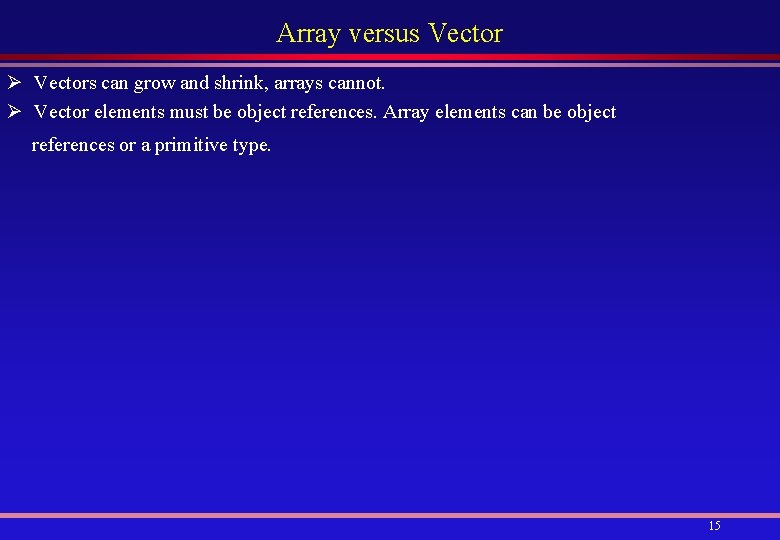# Dynamic Arrays Why Dynamic Arrays A Dynamic Array

• Slides: 15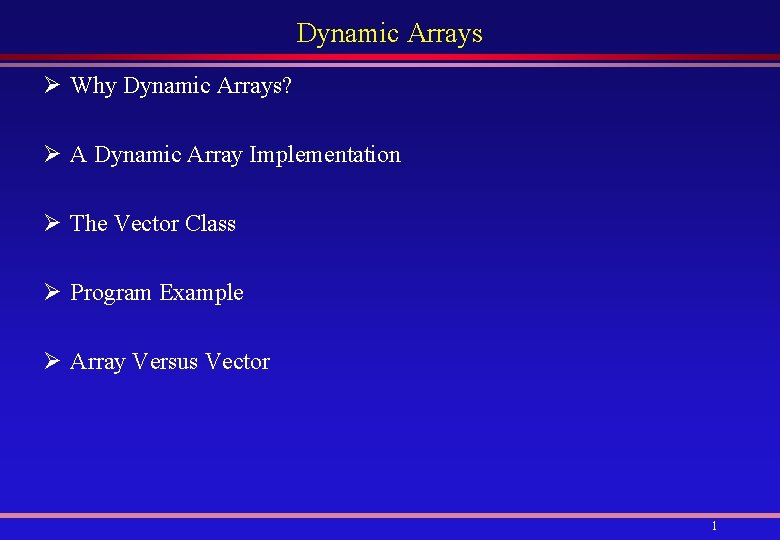Dynamic Arrays Ø Why Dynamic Arrays? Ø A Dynamic Array Implementation Ø The Vector Class Ø Program Example Ø Array Versus Vector 1Why Dynamic Arrays? Ø A problem with arrays is that their size must be fixed at creation. Ø Thus, once an array of size n is declared, it cannot be extended to hold more than n elements. Ø But the programmer may not know the size required. Ø Is there a way out? Ø Yes, Java provides the Vector class in the java. util package that can grow dynamically as needed. Ø To understand how it works, we shall implement a similar class. 2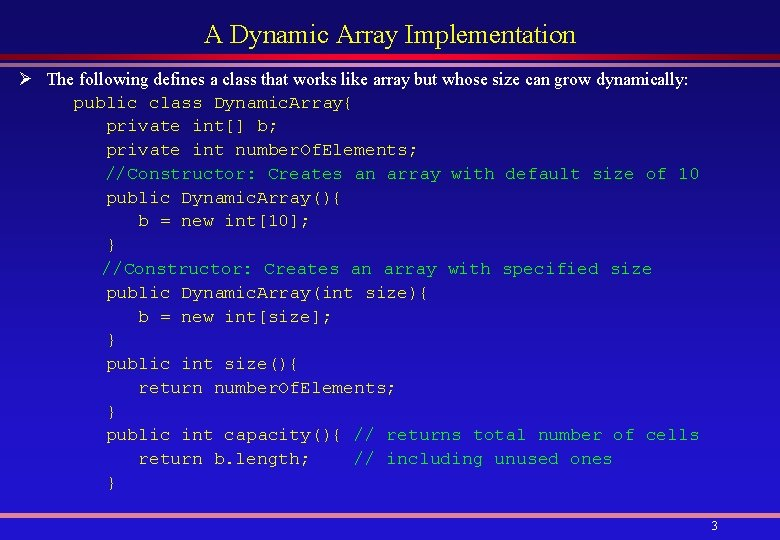A Dynamic Array Implementation Ø The following defines a class that works like array but whose size can grow dynamically: public class Dynamic. Array{ private int[] b; private int number. Of. Elements; //Constructor: Creates an array with default size of 10 public Dynamic. Array(){ b = new int; } //Constructor: Creates an array with specified size public Dynamic. Array(int size){ b = new int[size]; } public int size(){ return number. Of. Elements; } public int capacity(){ // returns total number of cells return b. length; // including unused ones } 3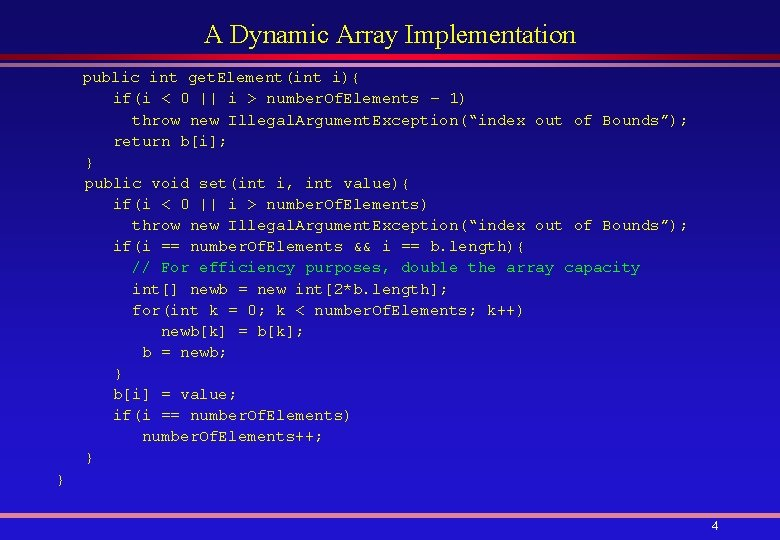A Dynamic Array Implementation public int get. Element(int i){ if(i < 0 || i > number. Of. Elements – 1) throw new Illegal. Argument. Exception(“index out of Bounds”); return b[i]; } public void set(int i, int value){ if(i < 0 || i > number. Of. Elements) throw new Illegal. Argument. Exception(“index out of Bounds”); if(i == number. Of. Elements && i == b. length){ // For efficiency purposes, double the array capacity int[] newb = new int[2*b. length]; for(int k = 0; k < number. Of. Elements; k++) newb[k] = b[k]; b = newb; } b[i] = value; if(i == number. Of. Elements) number. Of. Elements++; } } 4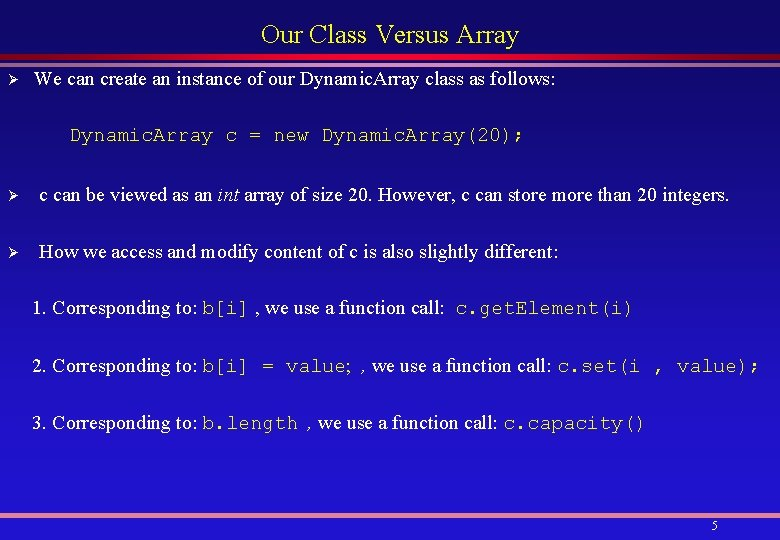Our Class Versus Array Ø We can create an instance of our Dynamic. Array class as follows: Dynamic. Array c = new Dynamic. Array(20); Ø c can be viewed as an int array of size 20. However, c can store more than 20 integers. Ø How we access and modify content of c is also slightly different: 1. Corresponding to: b[i] , we use a function call: c. get. Element(i) 2. Corresponding to: b[i] = value; , we use a function call: c. set(i , value); 3. Corresponding to: b. length , we use a function call: c. capacity() 5The Vector Class Ø Vector is similar to our Dynamic. Array, but it has much more. Ø It has the following Constructors: Vector() Creates a vector of size 10, It doubles the capacity when exhausted. Vector(int initial. Capacity) Creates a vector of size initial. Capacity, It doubles the capacity when exhausted. Vector(int initial. Capacity, int increment) Creates a vector of size initial. Capacity, increases by increment when the capacity is exhausted. Ø To Create a vector with an initial capacity of 20 elements: Vector v = new Vector(20); 6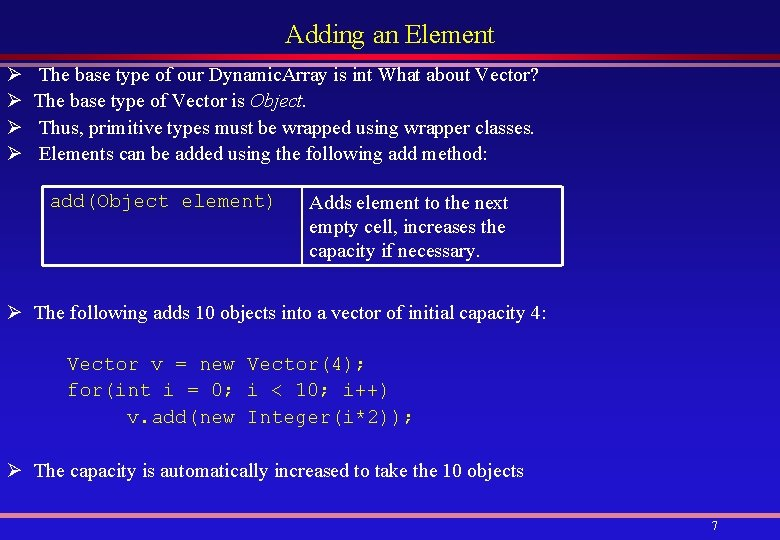Adding an Element Ø Ø The base type of our Dynamic. Array is int What about Vector? The base type of Vector is Object. Thus, primitive types must be wrapped using wrapper classes. Elements can be added using the following add method: add(Object element) Adds element to the next empty cell, increases the capacity if necessary. Ø The following adds 10 objects into a vector of initial capacity 4: Vector v = new Vector(4); for(int i = 0; i < 10; i++) v. add(new Integer(i*2)); Ø The capacity is automatically increased to take the 10 objects 7Adding an Element (cont’d) Ø The following add method can also be used to add an element: add(int index, Object element) Adds element at index, shifts element at index and beyond, if any, by 1. Increases capacity if necessary. If index < 0 or index > size, it throws Index. Out. Of. Bounds. Exception. Ø The following adds 6 objects into a vector, it then inserts an Integer object with value of 20 at index 3: Vector v = new Vector(4); for(int i = 0; i < 6; i++) v. add(new Integer(i*2)); v. add(3, new Integer(20)); v 0 1 2 3 4 5 6 0 2 4 20 6 8 10 reference Ø Note that this method does not allow an empty cell in-between: v. add(8, new Integer(100)); // Run-time error, cell 7 will be empty 8 7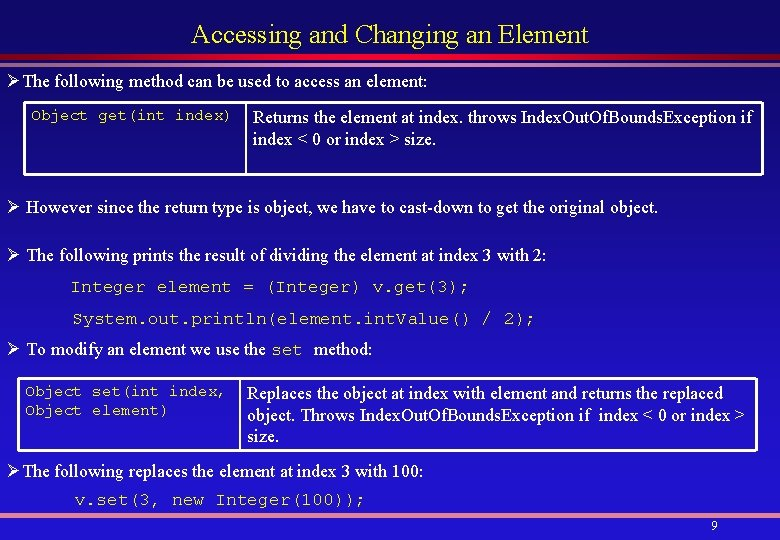Accessing and Changing an Element ØThe following method can be used to access an element: Object get(int index) Returns the element at index. throws Index. Out. Of. Bounds. Exception if index < 0 or index > size. Ø However since the return type is object, we have to cast-down to get the original object. Ø The following prints the result of dividing the element at index 3 with 2: Integer element = (Integer) v. get(3); System. out. println(element. int. Value() / 2); Ø To modify an element we use the set method: Object set(int index, Replaces the object at index with element and returns the replaced Object element) object. Throws Index. Out. Of. Bounds. Exception if index < 0 or index > size. ØThe following replaces the element at index 3 with 100: v. set(3, new Integer(100)); 9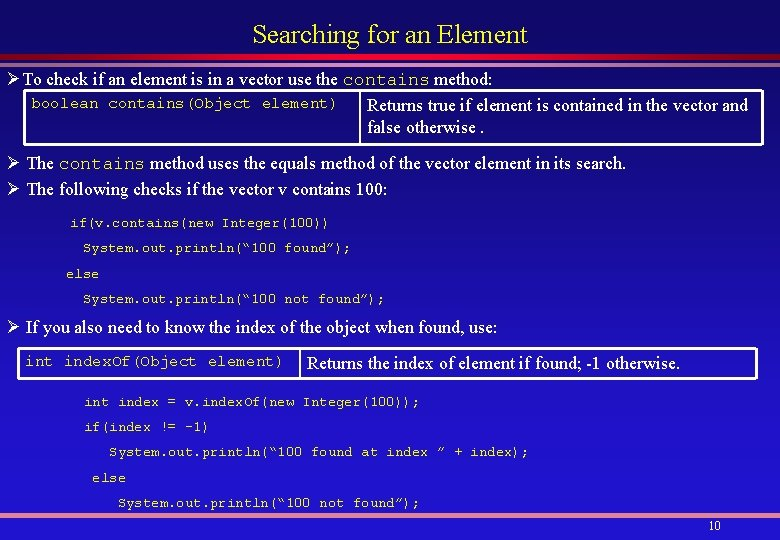Searching for an Element ØTo check if an element is in a vector use the contains method: boolean contains(Object element) Returns true if element is contained in the vector and false otherwise. Ø The contains method uses the equals method of the vector element in its search. Ø The following checks if the vector v contains 100: if(v. contains(new Integer(100)) System. out. println(“ 100 found”); else System. out. println(“ 100 not found”); Ø If you also need to know the index of the object when found, use: int index. Of(Object element) Returns the index of element if found; -1 otherwise. int index = v. index. Of(new Integer(100)); if(index != -1) System. out. println(“ 100 found at index ” + index); else System. out. println(“ 100 not found”); 10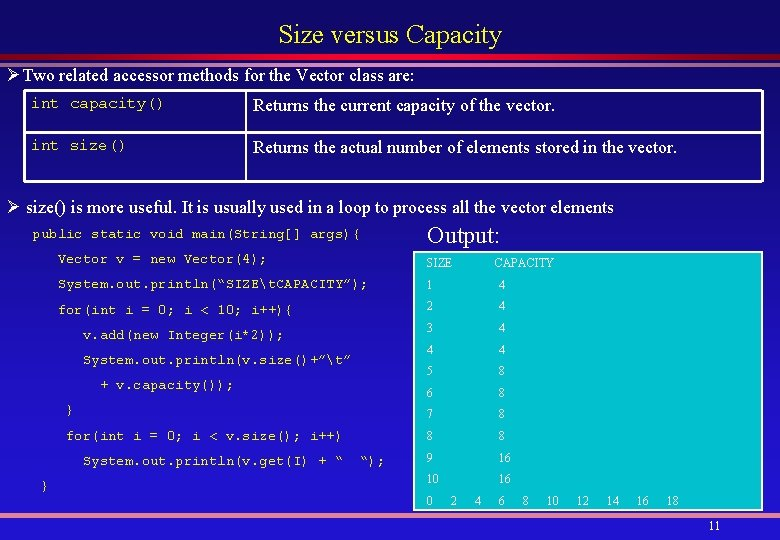Size versus Capacity ØTwo related accessor methods for the Vector class are: int capacity() Returns the current capacity of the vector. int size() Returns the actual number of elements stored in the vector. Ø size() is more useful. It is usually used in a loop to process all the vector elements public static void main(String[] args){ Output: Vector v = new Vector(4); SIZE CAPACITY System. out. println(“SIZEt. CAPACITY”); 1 4 for(int i = 0; i < 10; i++){ 2 4 v. add(new Integer(i*2)); 3 4 4 4 5 8 6 8 } 7 8 for(int i = 0; i < v. size(); i++) 8 8 System. out. println(v. get(I) + “ “); 9 16 } 10 16 System. out. println(v. size()+”t” + v. capacity()); 0 2 4 6 8 10 12 14 16 18 11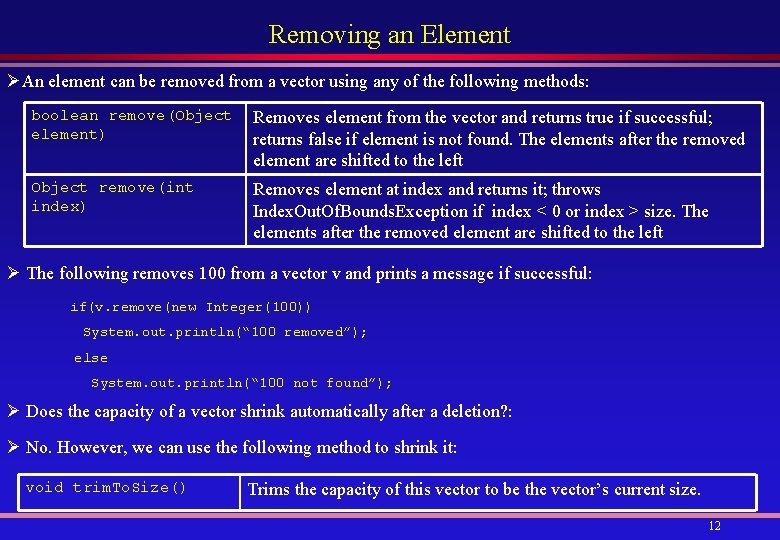Removing an Element ØAn element can be removed from a vector using any of the following methods: boolean remove(Object Removes element from the vector and returns true if successful; element) returns false if element is not found. The elements after the removed element are shifted to the left Object remove(int index) Removes element at index and returns it; throws Index. Out. Of. Bounds. Exception if index < 0 or index > size. The elements after the removed element are shifted to the left Ø The following removes 100 from a vector v and prints a message if successful: if(v. remove(new Integer(100)) System. out. println(“ 100 removed”); else System. out. println(“ 100 not found”); Ø Does the capacity of a vector shrink automatically after a deletion? : Ø No. However, we can use the following method to shrink it: void trim. To. Size() Trims the capacity of this vector to be the vector’s current size. 12